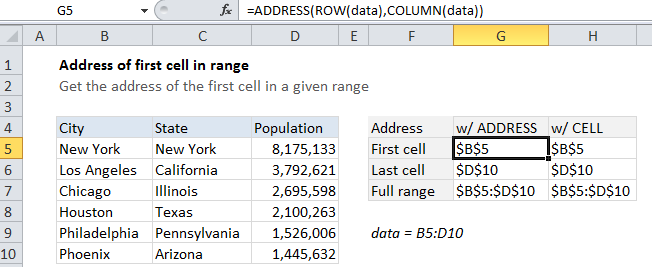## Excel Office

Excel How Tos, Tutorials, Tips & Tricks, Shortcuts

# How to get address of first cell in range in Excel

To get the address of the first cell in a named range, you can use the ADDRESS function together with the ROW and COLUMN functions. See example below;

Note: The ADDRESS function builds an address based on a row and column number.

## Formula

`=ADDRESS(ROW(range),COLUMN(range))`## Explanation

In the example shown, the formula in G5 is:

`=ADDRESS(ROW(data),COLUMN(data))`

### How this formula works

Working from the inside out, the ROW function returns an array of absolute* row numbers for a given range, and the COLUMN function returns an array of column numbers.

Worked Example:   Automatically fill series of cells in Excel using AutoFill

The ADDRESS function then assembles and returns an array of addresses. When entered in a single cell, just the item from the array is displayed, which is the address corresponding to the first cell in a range.

Worked Example:   Get work hours between dates custom schedule in Excel

* By absolute, I mean numbers that track to the entire worksheet, not numbers relative to the range itself.

### CELL function alternative

Note that the the CELL function can be volatile. If you add it to a worksheet, you’ll notice that you are prompted to save even when you’ve made no changes.

Worked Example:   How to use Excel COLUMN Function

You can also use the CELL function to get the address of the first cell in a range with this formula:

`=CELL("address",data)`

In this case, we just pass the range into the CELL function with “address”, which returns the address of the first cell in the range.